DAY 20
0
AI & Data

一、資料正規化(Data Normalization)

讀入圖片

import cv2
img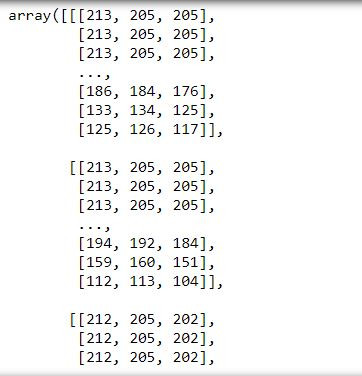將陣列中的像素值全部除以255(最大像素值)

img=img/255.0
img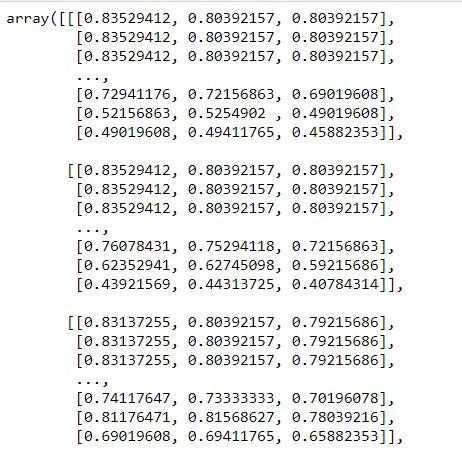二、資料增強(Data Augmentation)

具體作法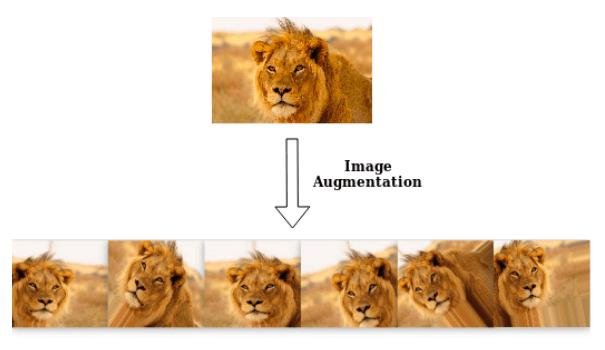如何使用

conda install tensorflow==2.5.0

載入套件

from tensorflow.keras.preprocessing.image import ImageDataGenerator

定義其參數

datagen=
ImageDataGenerator(featurewise_center=False,
samplewise_center=False,
featurewise_std_normalization=False,
samplewise_std_normalization=False,
zca_whitening=False,
zca_epsilon=1e-6,
rotation_range=0.,
width_shift_range=0.,
height_shift_range=0.,
shear_range=0.,
zoom_range=0.,
channel_shift_range=0.,
fill_mode='nearest',
cval=0.,
horizontal_flip=False,
vertical_flip=False,
rescale=None,
preprocessing_function=None,
data_format=K.image_data_format())

參數說明

featurewise_center：去中心值，使數據集均值為0

featurewise_std_normalization：使每個輸入樣本除以自身標準差

samplewise_center：去中心值，使輸入樣本均值為0

samplewise_std_normalization：使每個輸入樣本除以自身標準差(只考慮自身圖片)

zca_whtening：一種PCA降維處理，減少圖片的冗餘信息，保留最重要的特徵

zca_epsilon：zca白話的值

rotation_range：使圖片隨機旋轉的角度，輸入一個值，演算法會使圖片在這個值區間隨機旋轉

width_shift_range：圖片水平平移的尺寸

height_shift_range：圖片垂直平移的尺寸

shear_range：隨機裁減的角度

zoom_range：隨機縮放大小

channel_shift_range：隨機改變圖片的顏色

fill_mode：圖片經處理後邊界以外的點的處理方式

horizontal_flip：隨機進行水平翻轉

vertical_flip：隨機進行垂直翻轉

rescale：對圖片的每個像素值乘上這個縮放值，可理解為圖片正規化

preprocessing_function：其他的前處理功能，可自行寫def定義或是使用套件提供的

1. flow_from_directory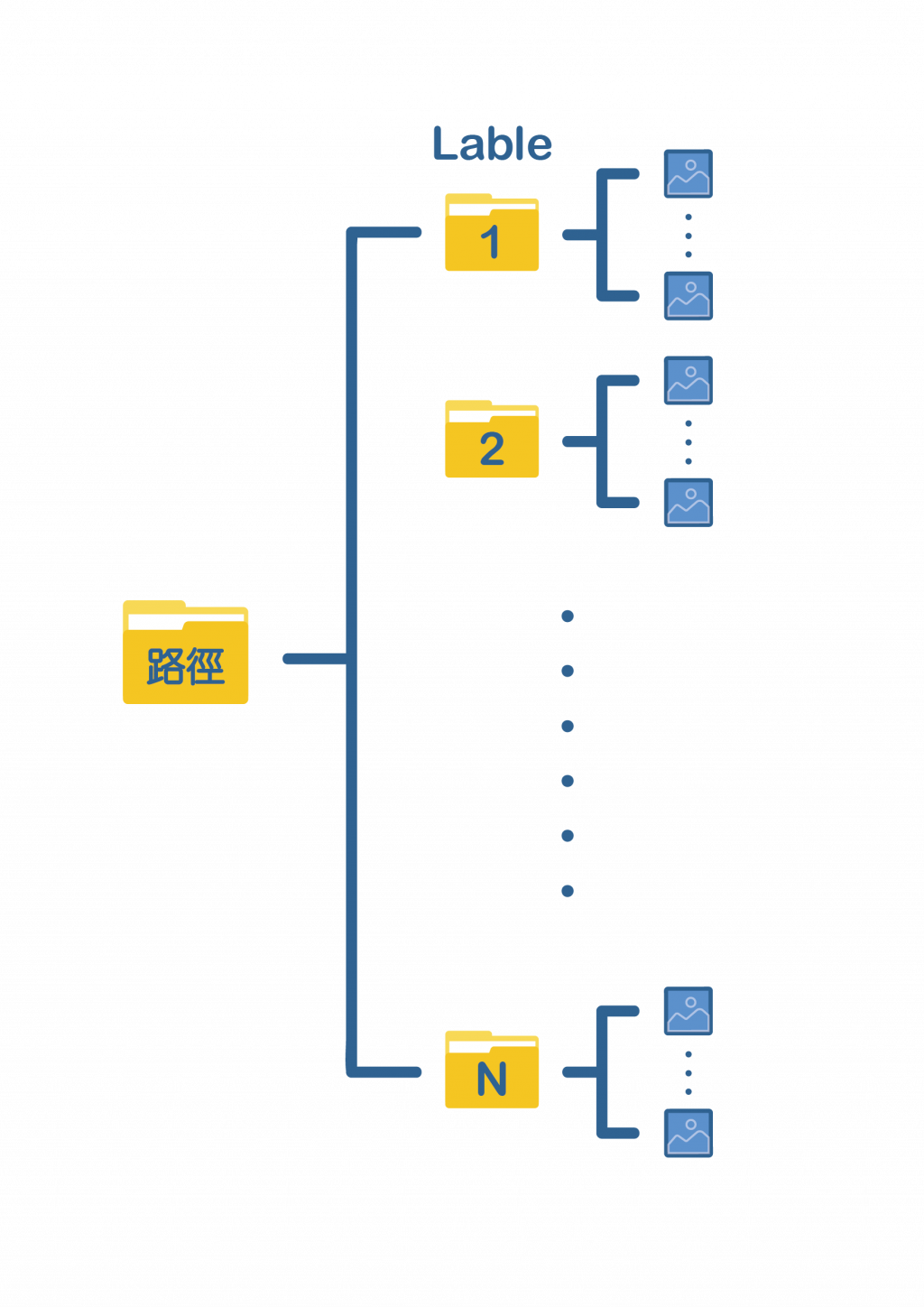程式碼

train_generator=
datagen.flow_from_dataframe(
directory,
labels="inferred",
label_mode="int",
class_names=None,
color_mode="rgb",
batch_size=32,
image_size=(256, 256),
shuffle=True,
seed=None,
validation_split=None,
subset=None,
interpolation="bilinear",
crop_to_aspect_ratio=False,
**kwargs
)

directory放置最上層資料夾的路徑，labels選擇"inferred"，其餘參數像是image_size、batch_size等皆可自行調整，詳細的參數說明可至Image data preprocessing查看。

2. flow_from_dataframe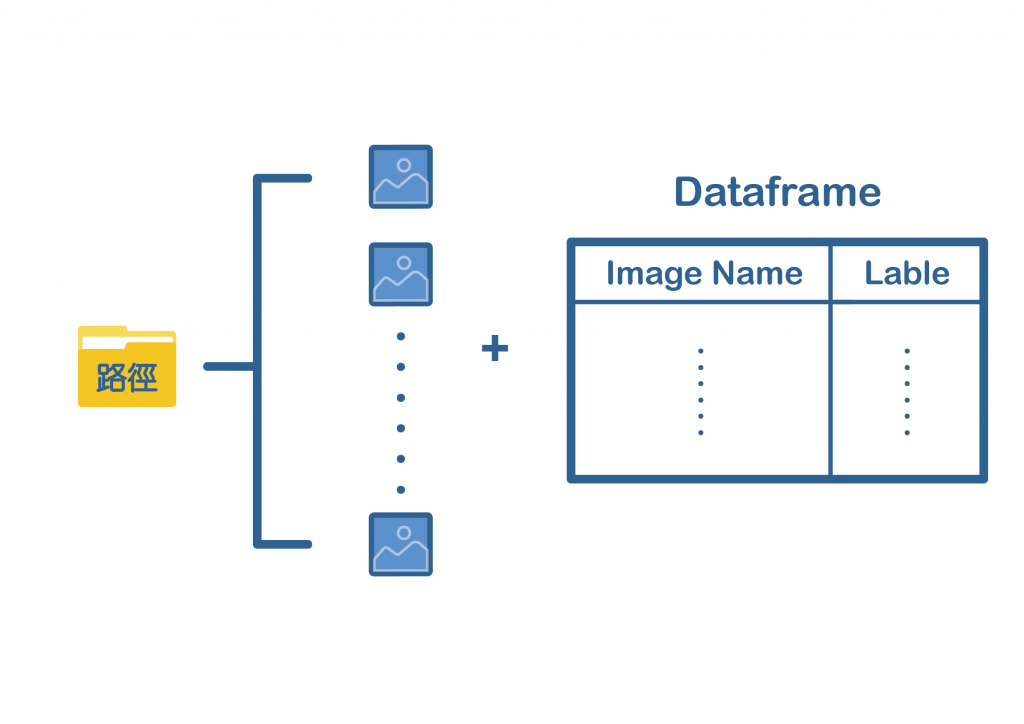程式碼

train_generator=
datagen.flow_from_dataframe(
dataframe,
directory=None,
x_col='filename',
y_col='class',
weight_col=None,
target_size=(256, 256),
color_mode='rgb',
classes=None,
class_mode='categorical',
batch_size=32,
shuffle=True,
seed=None,
save_to_dir=None,
save_prefix='',
save_format='png',
subset=None,
interpolation='nearest',
validate_filenames=True, **kwargs
)

Dataframe要放含有圖片名稱跟標籤的Dataframe，例如：模型訓練

fit_generator(
generator,
steps_per_epoch=None,
epochs=1, verbose=1,
callbacks=None,
validation_data=None,
validation_steps=None,
class_weight=None,
max_queue_size=10,
workers=1,
use_multiprocessing=False,
shuffle=True,
initial_epoch=0)